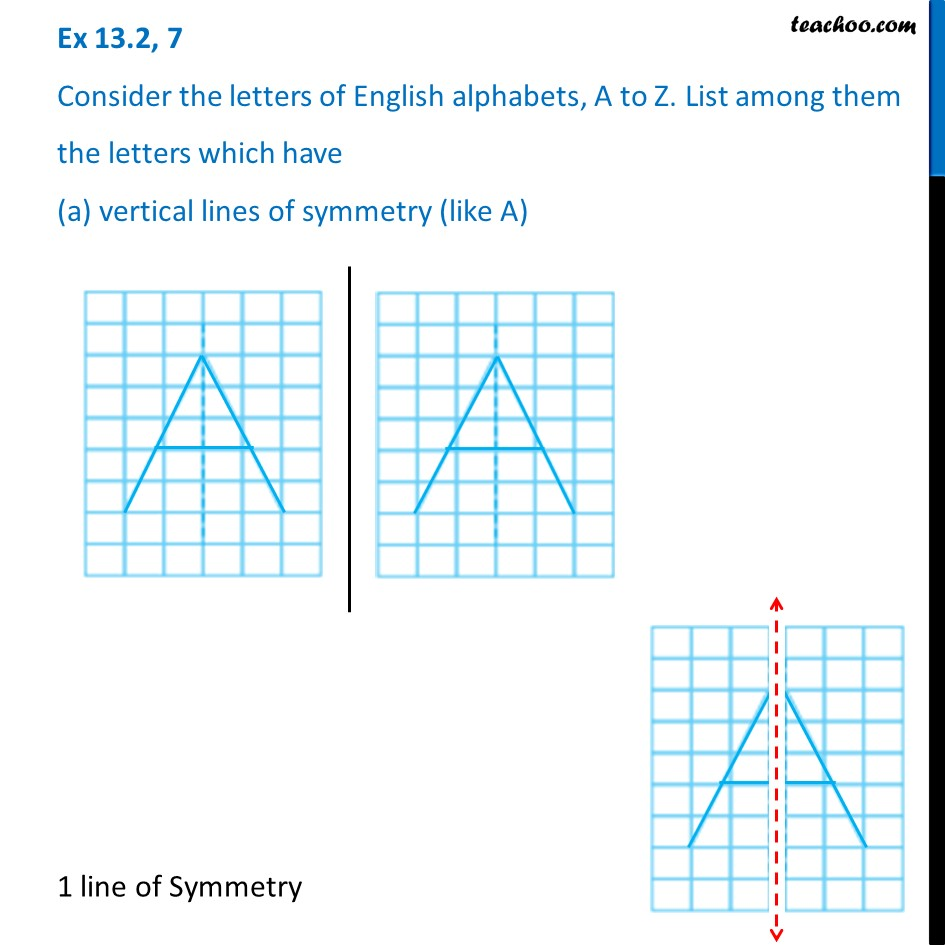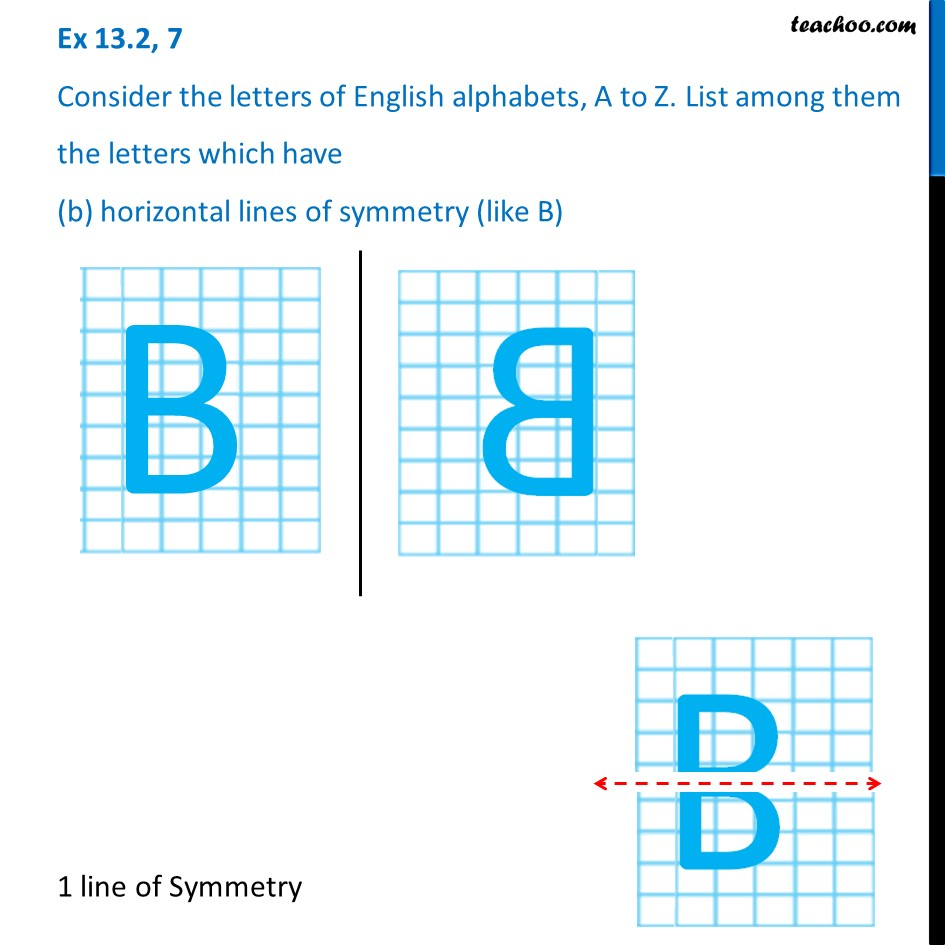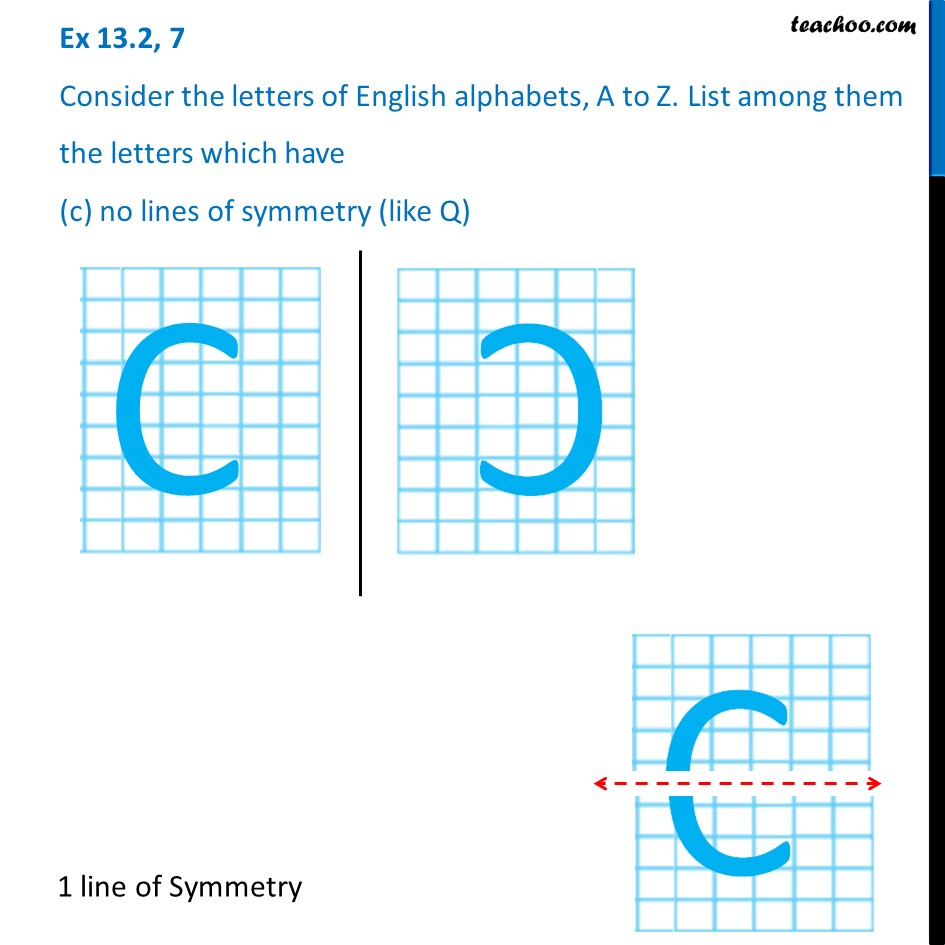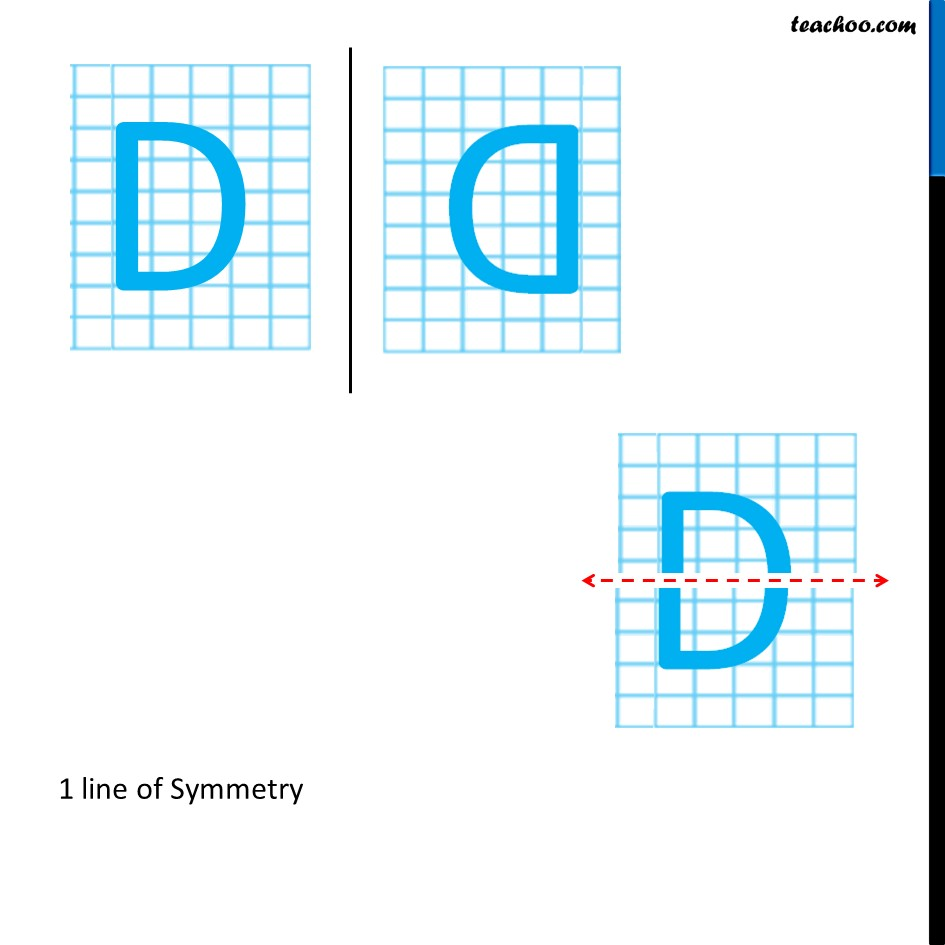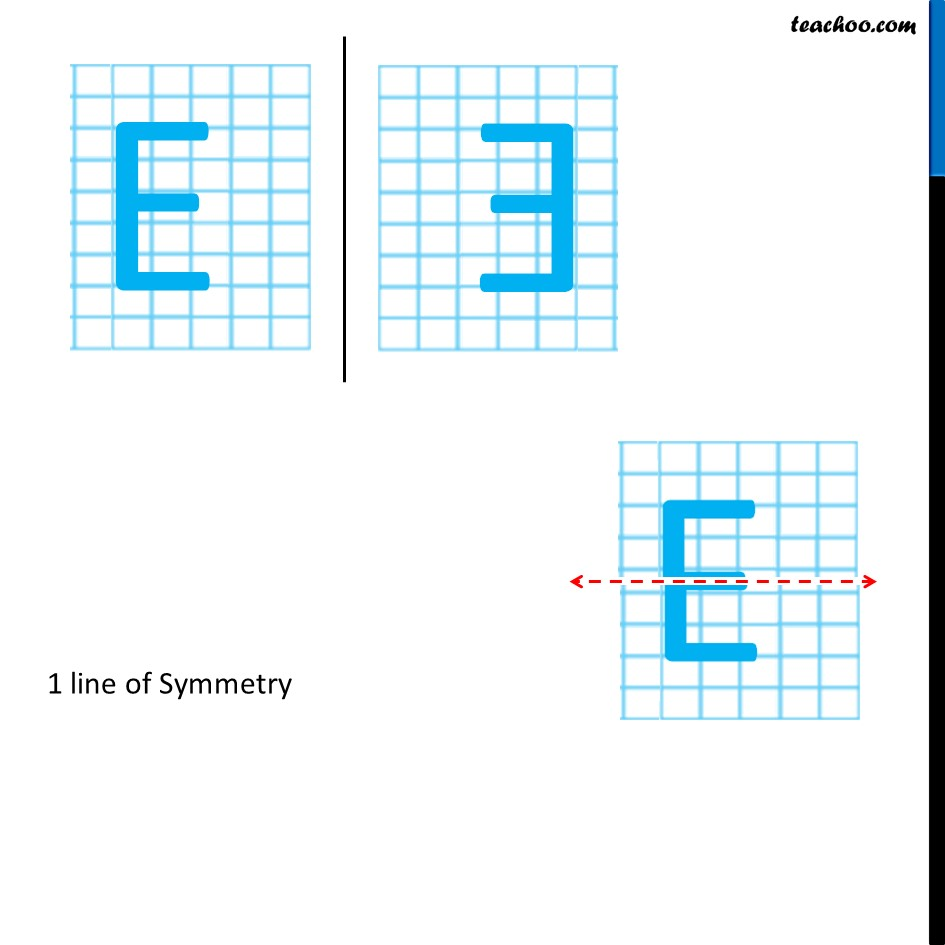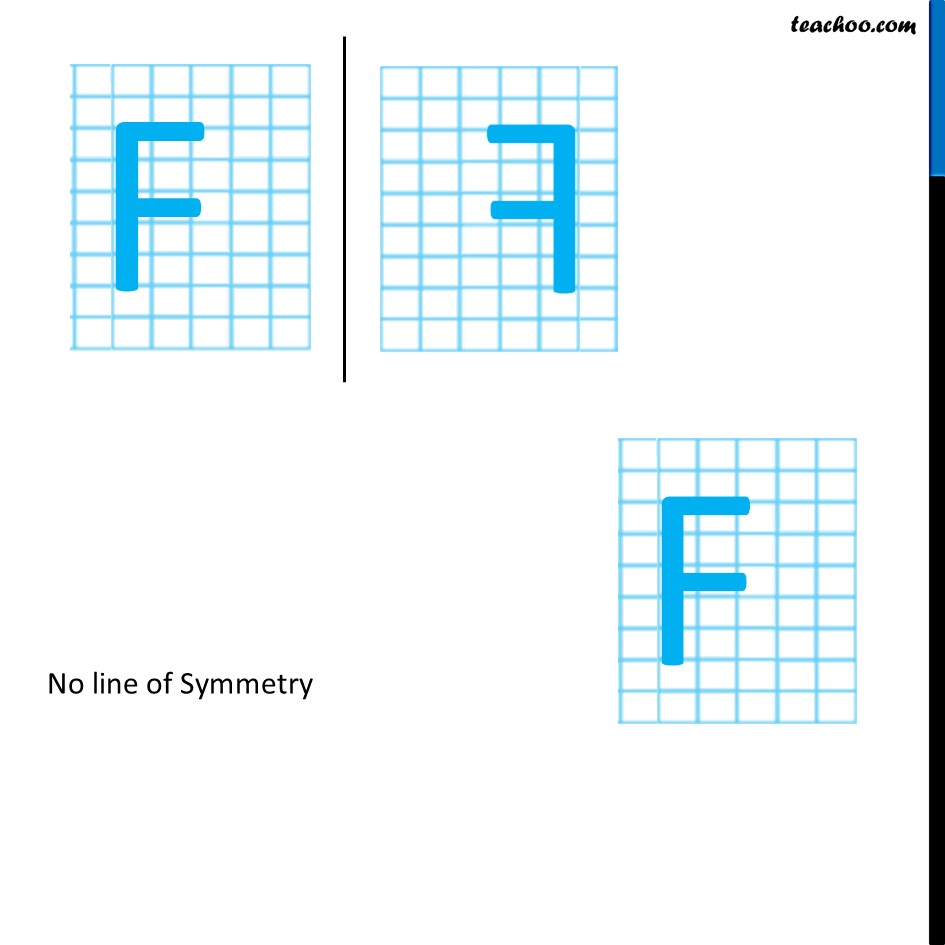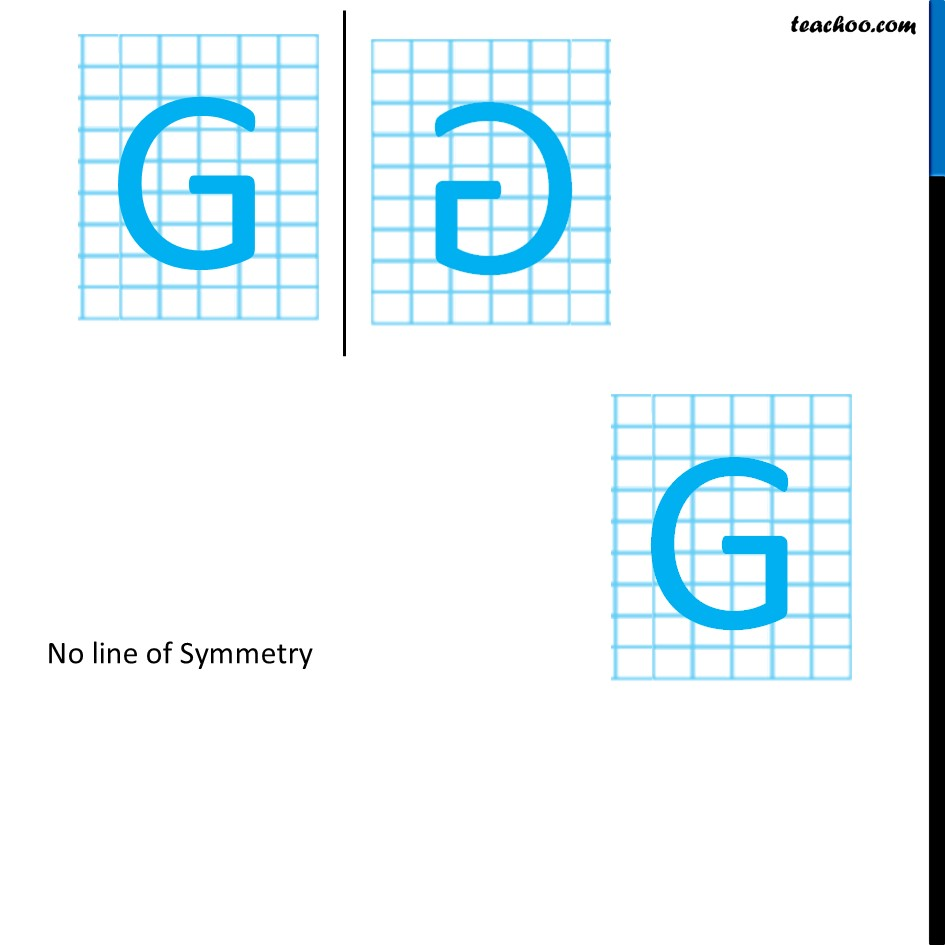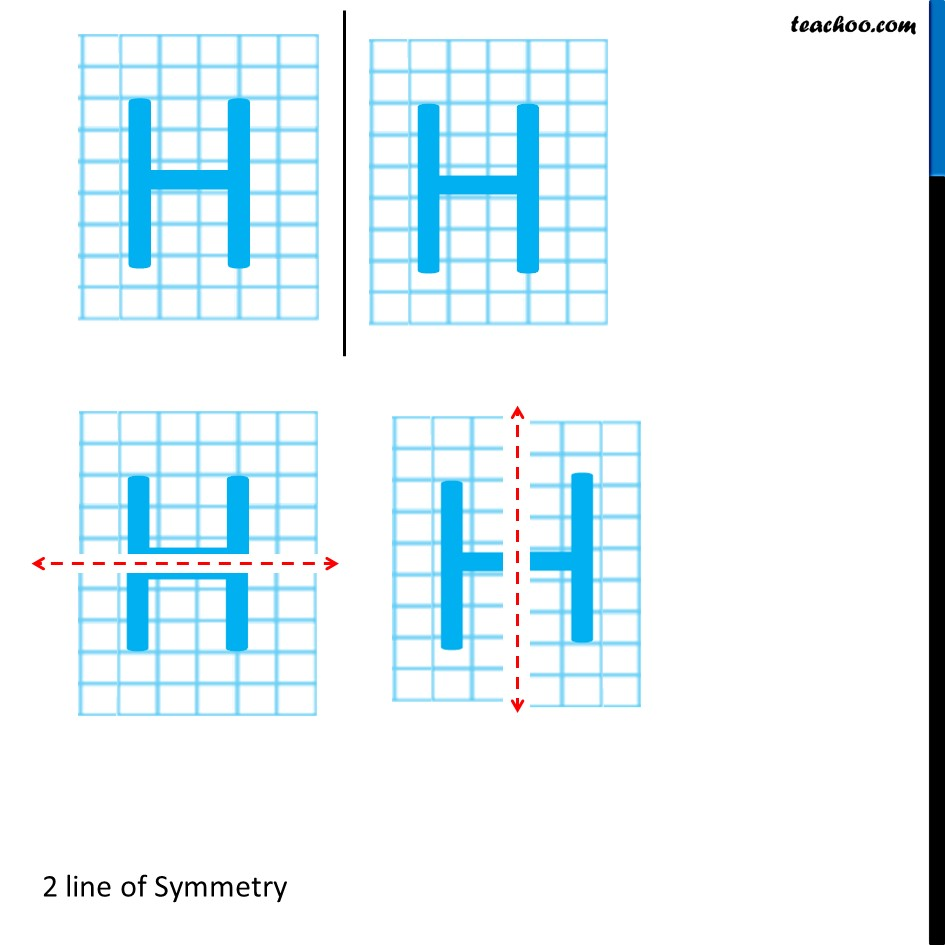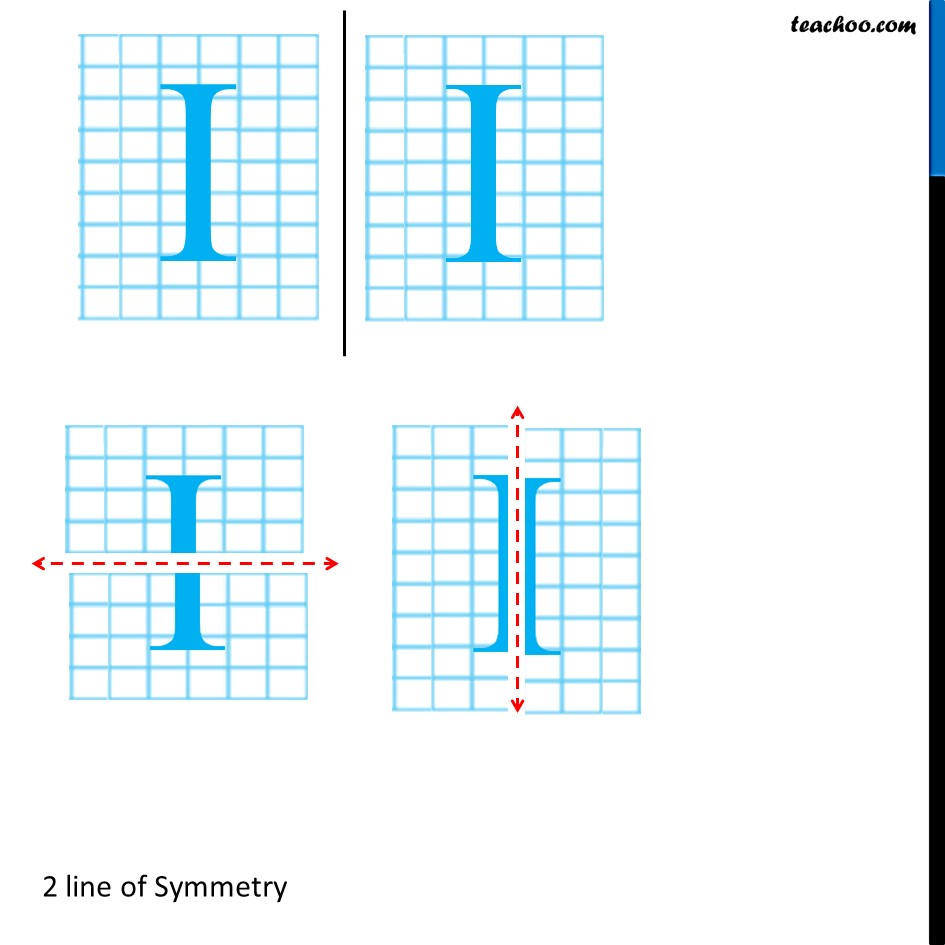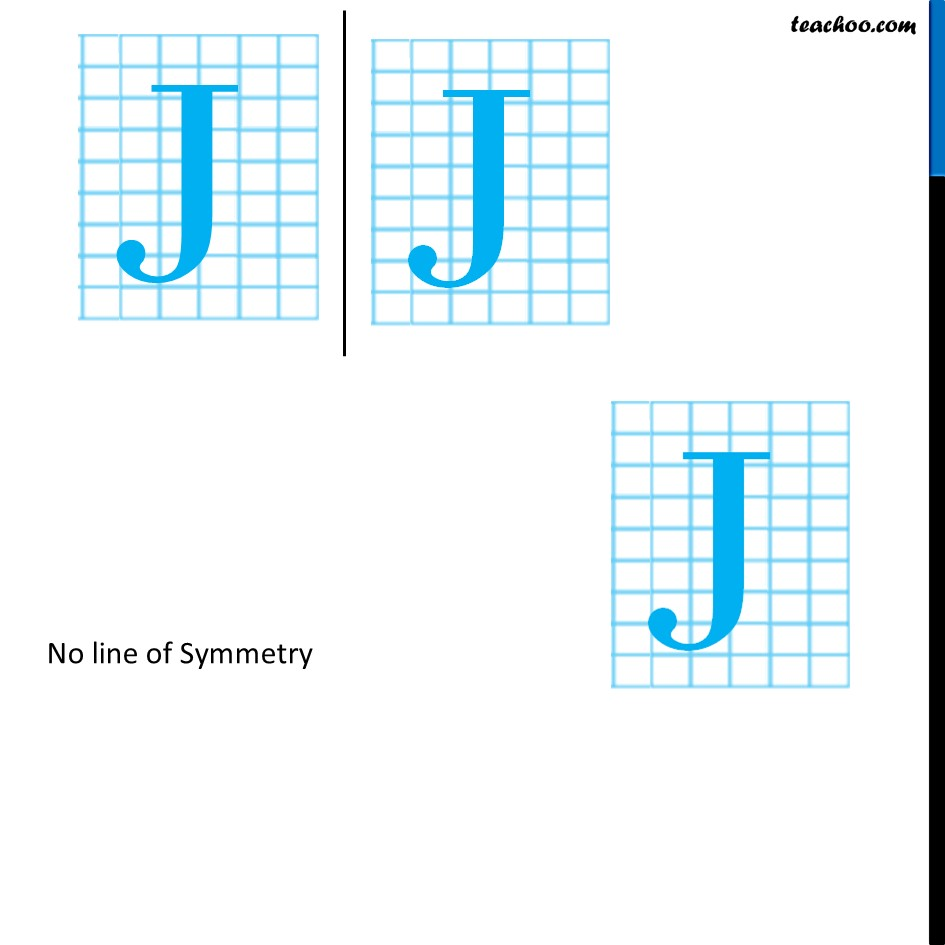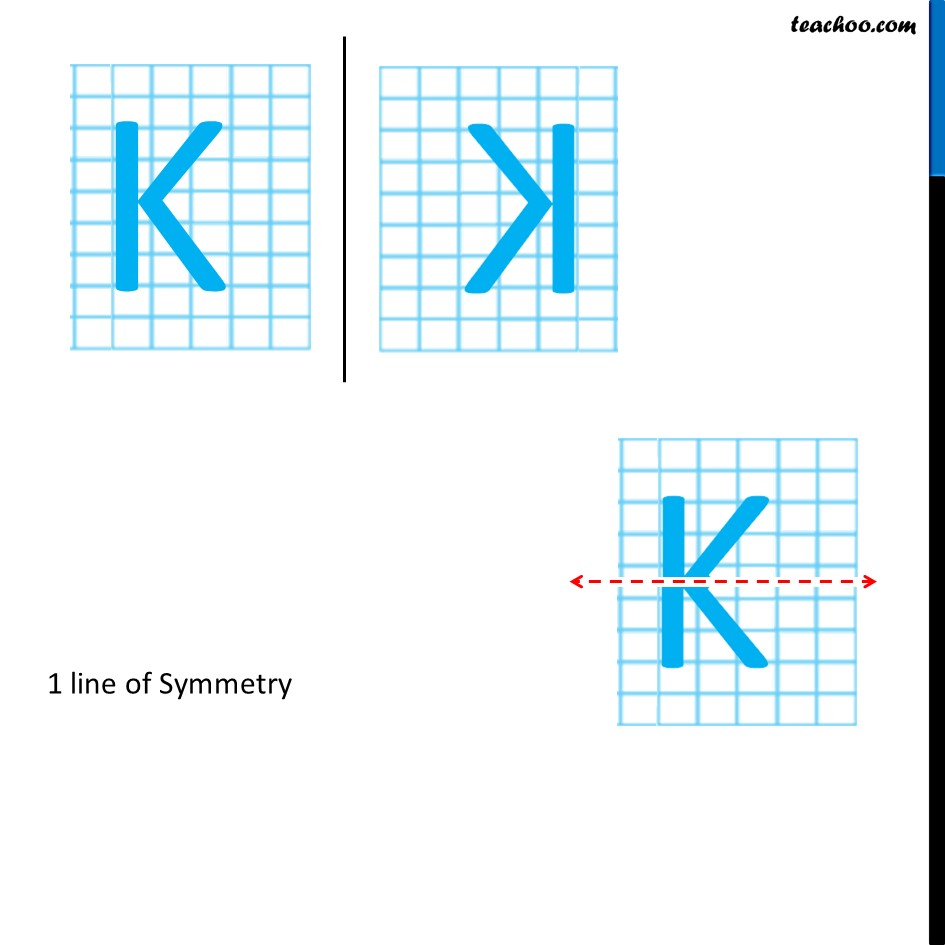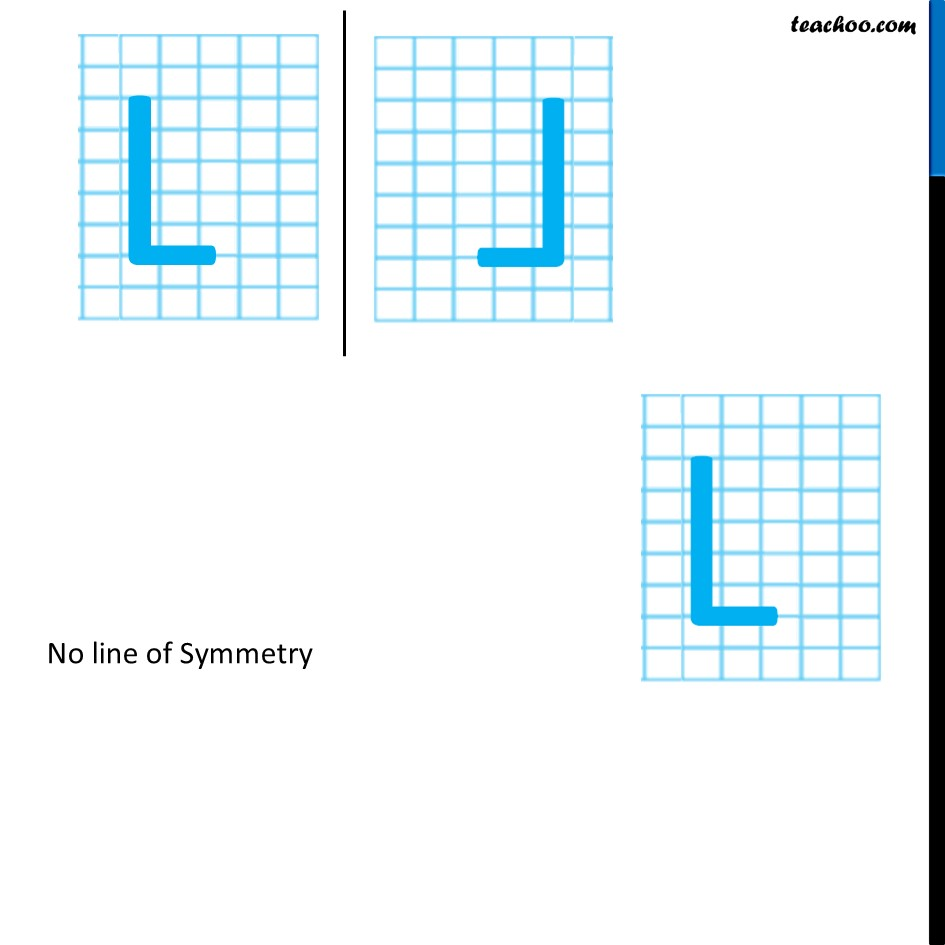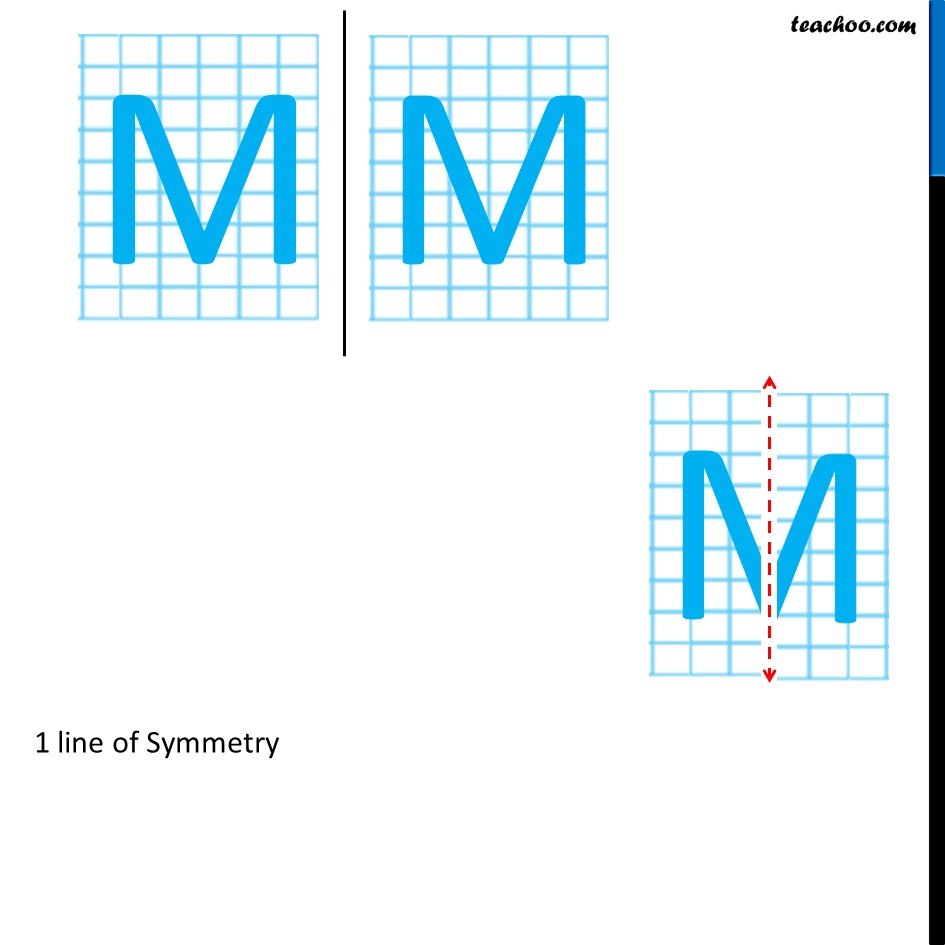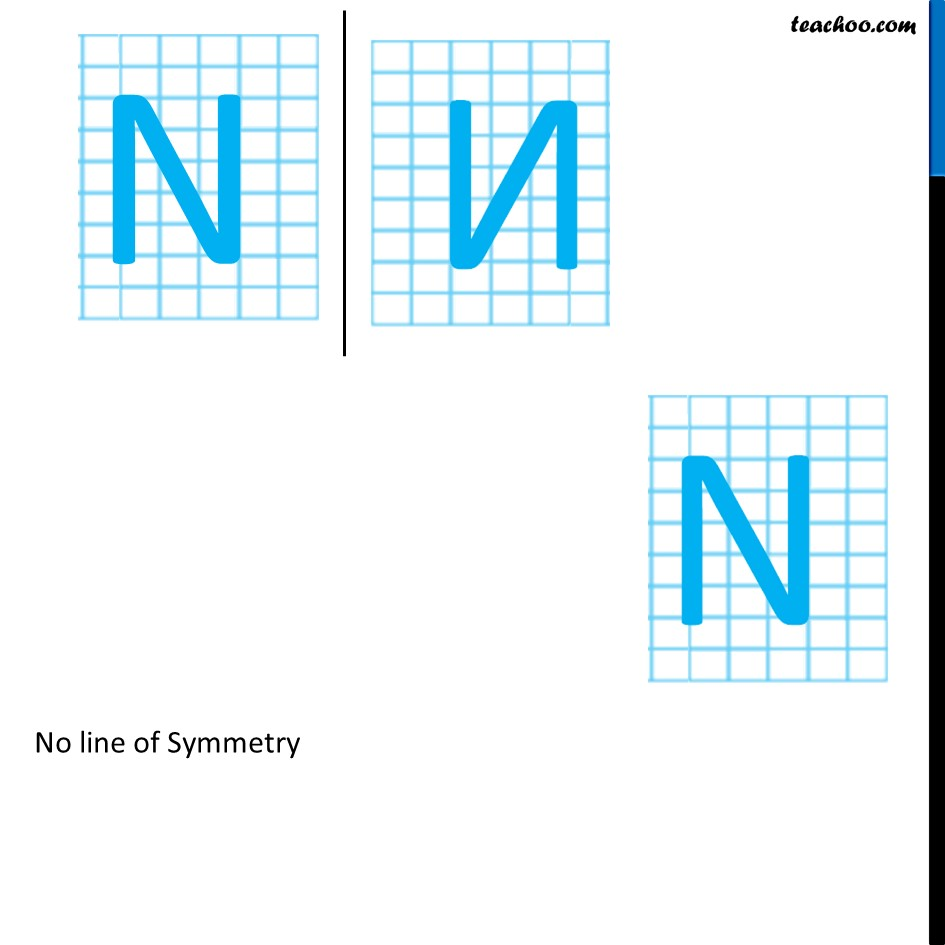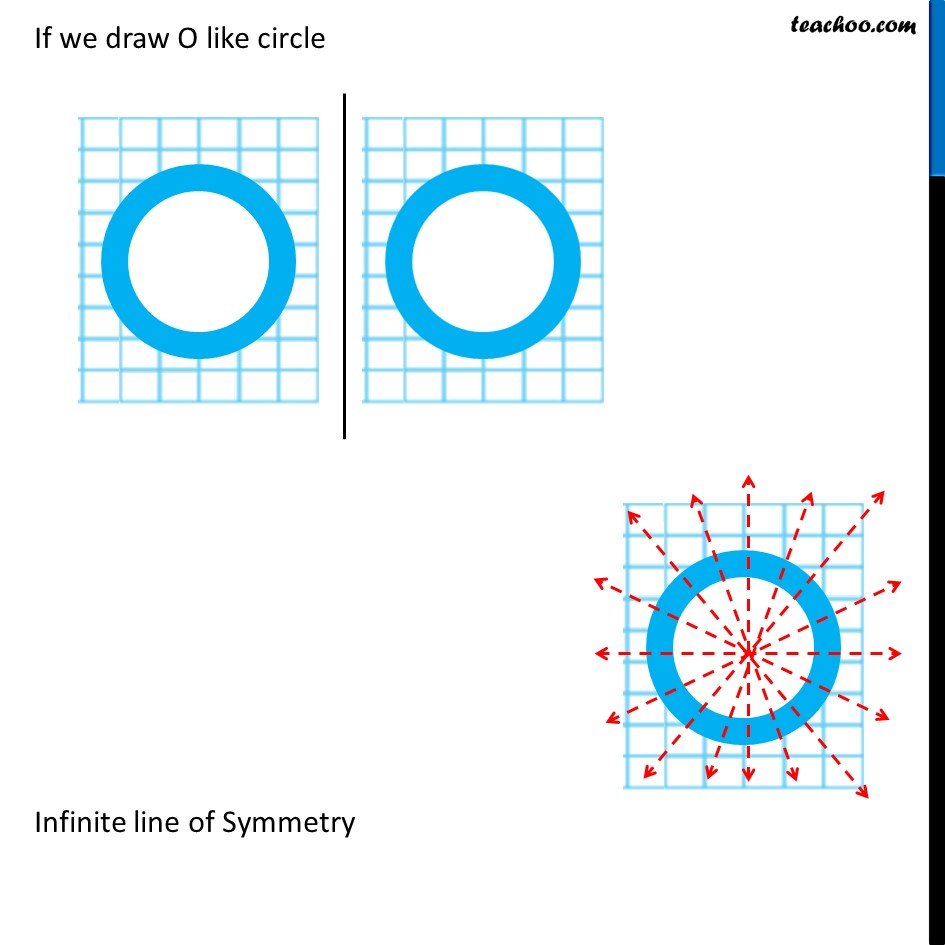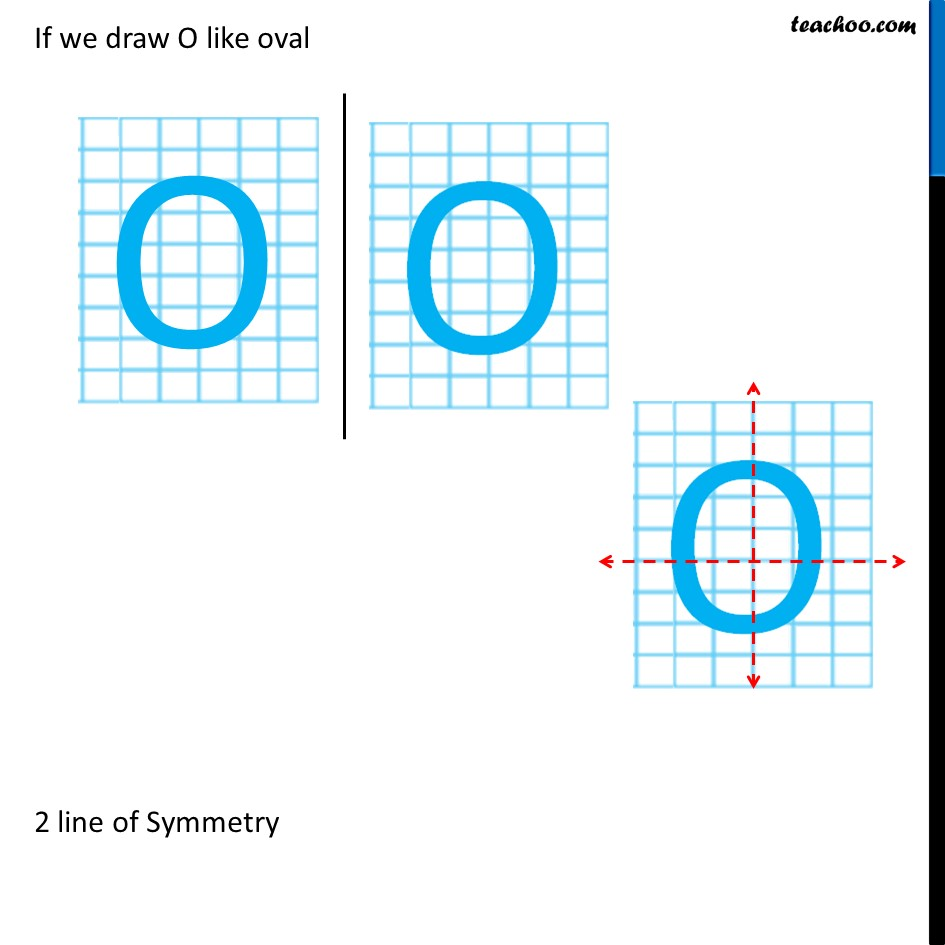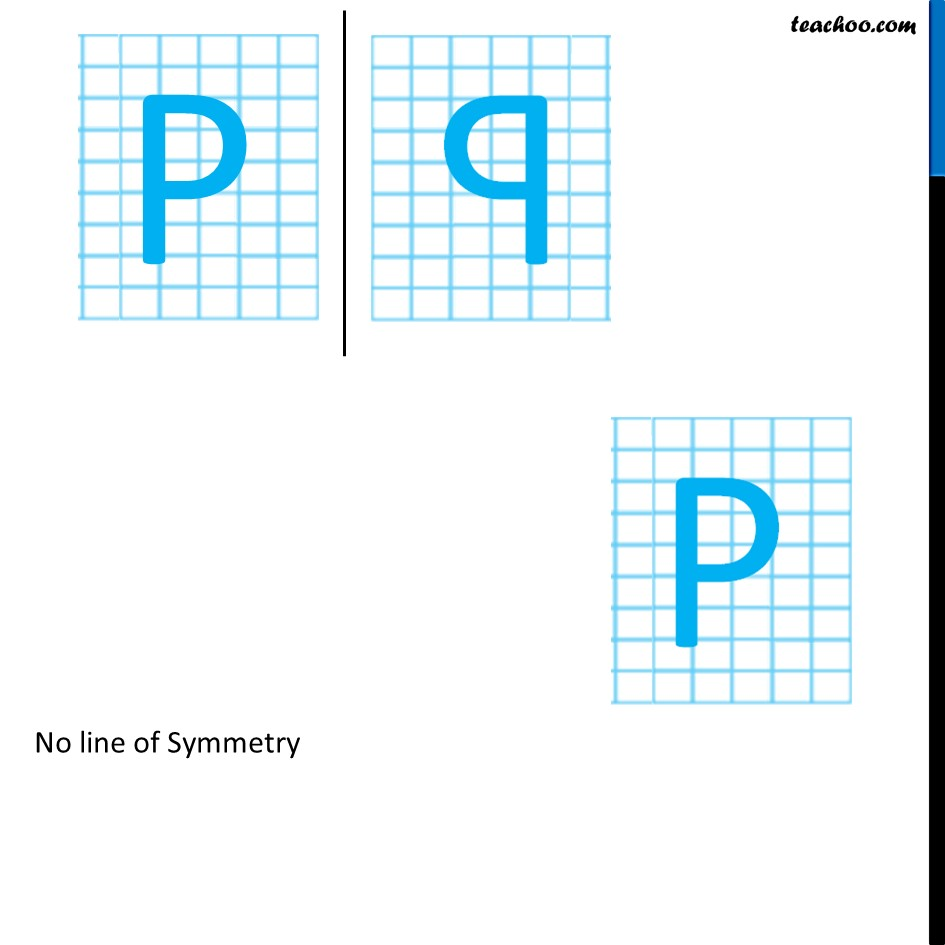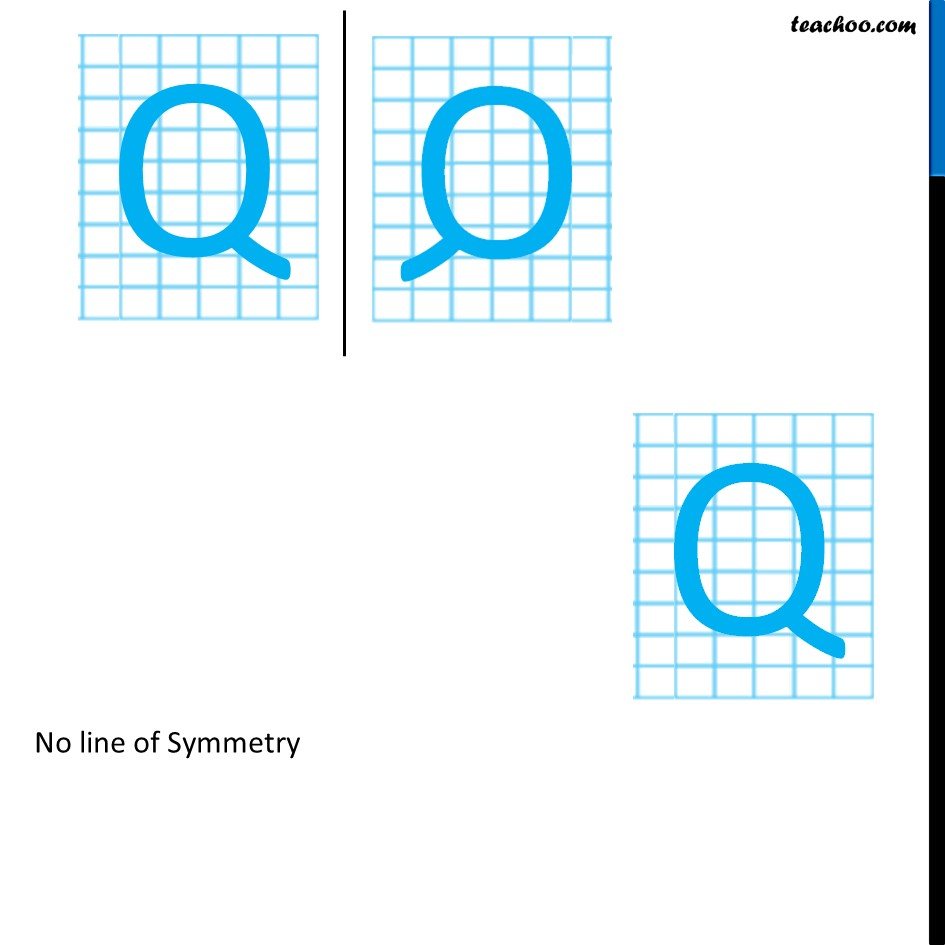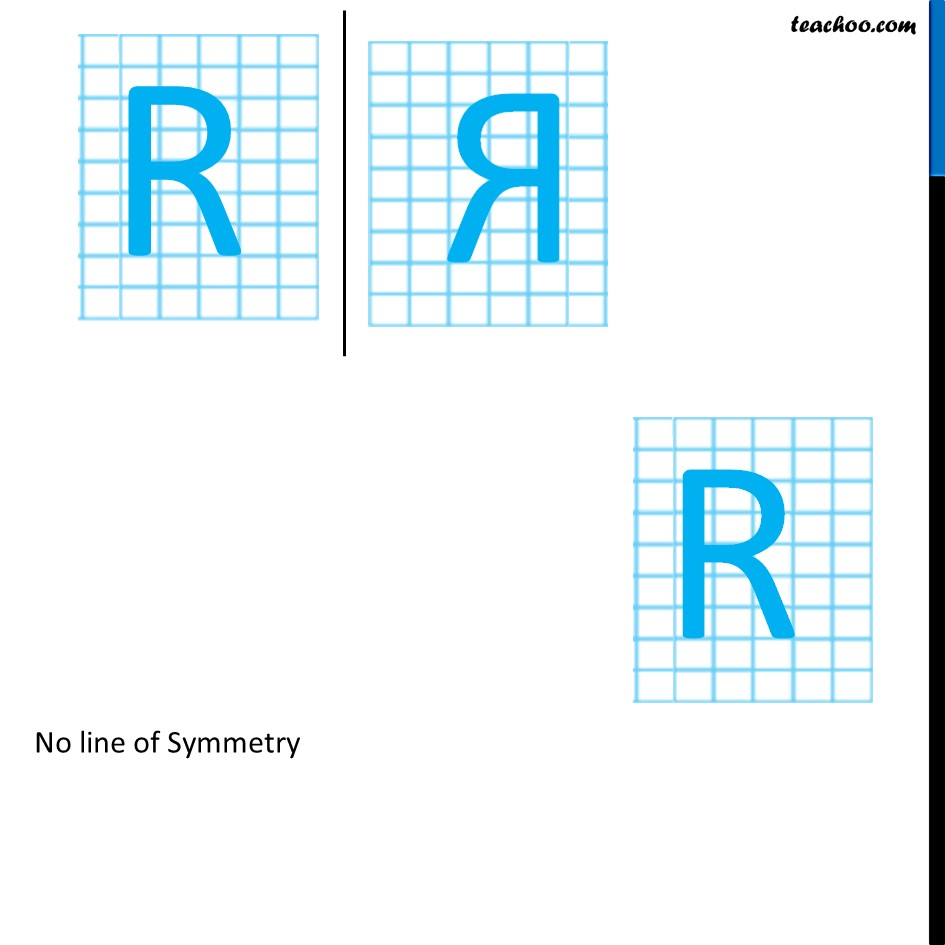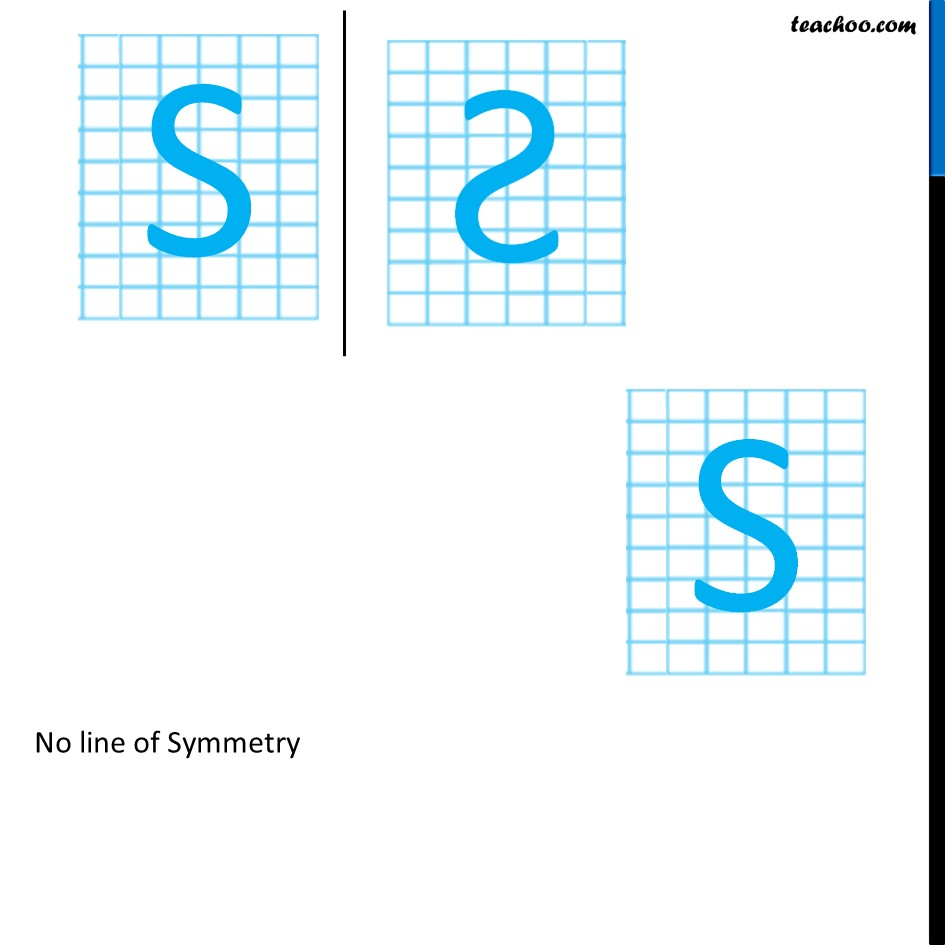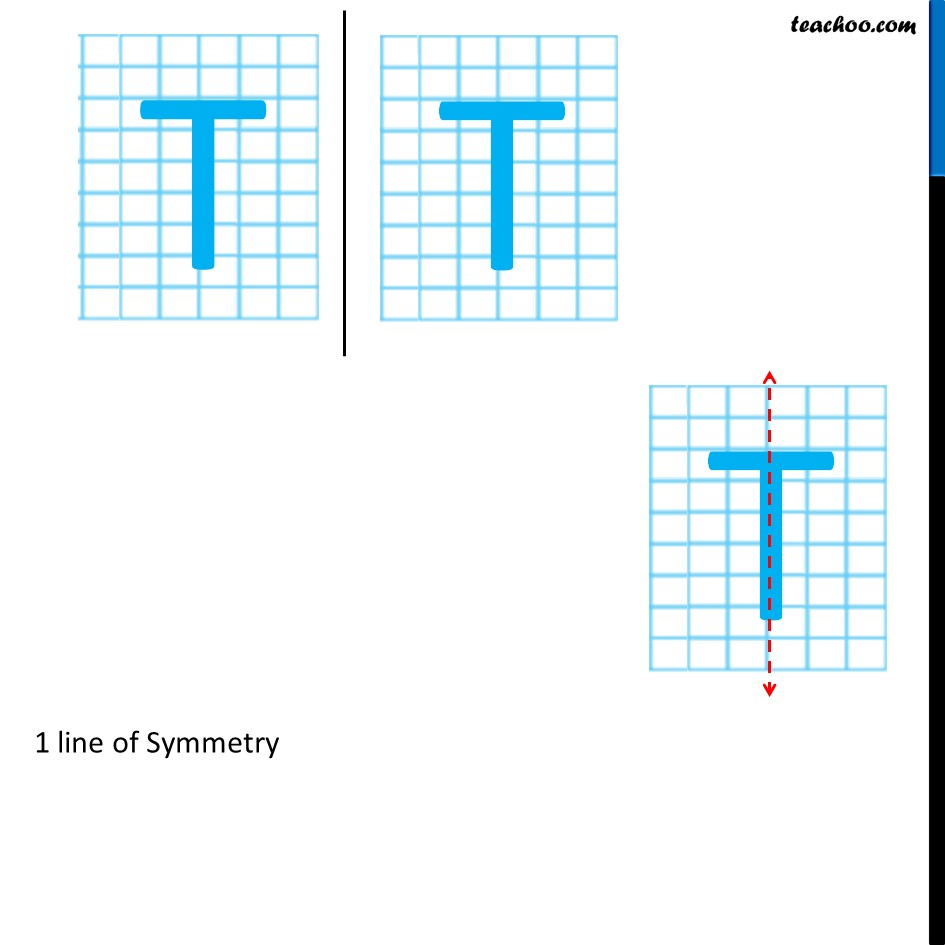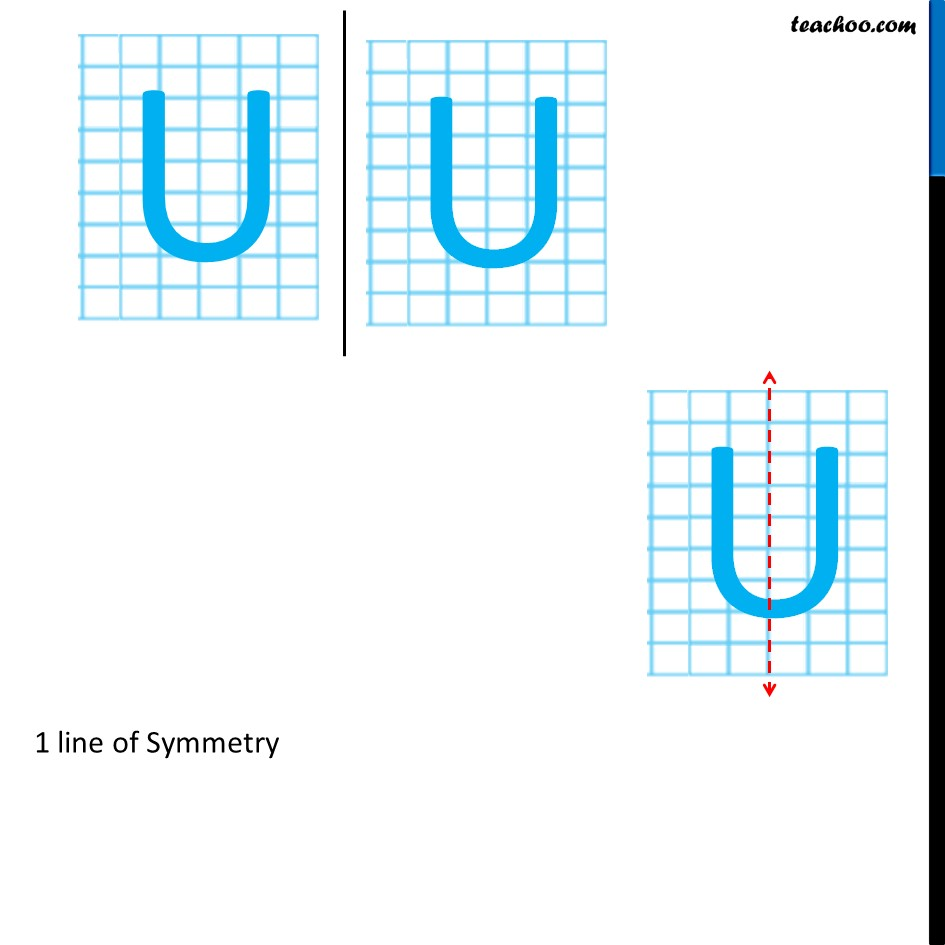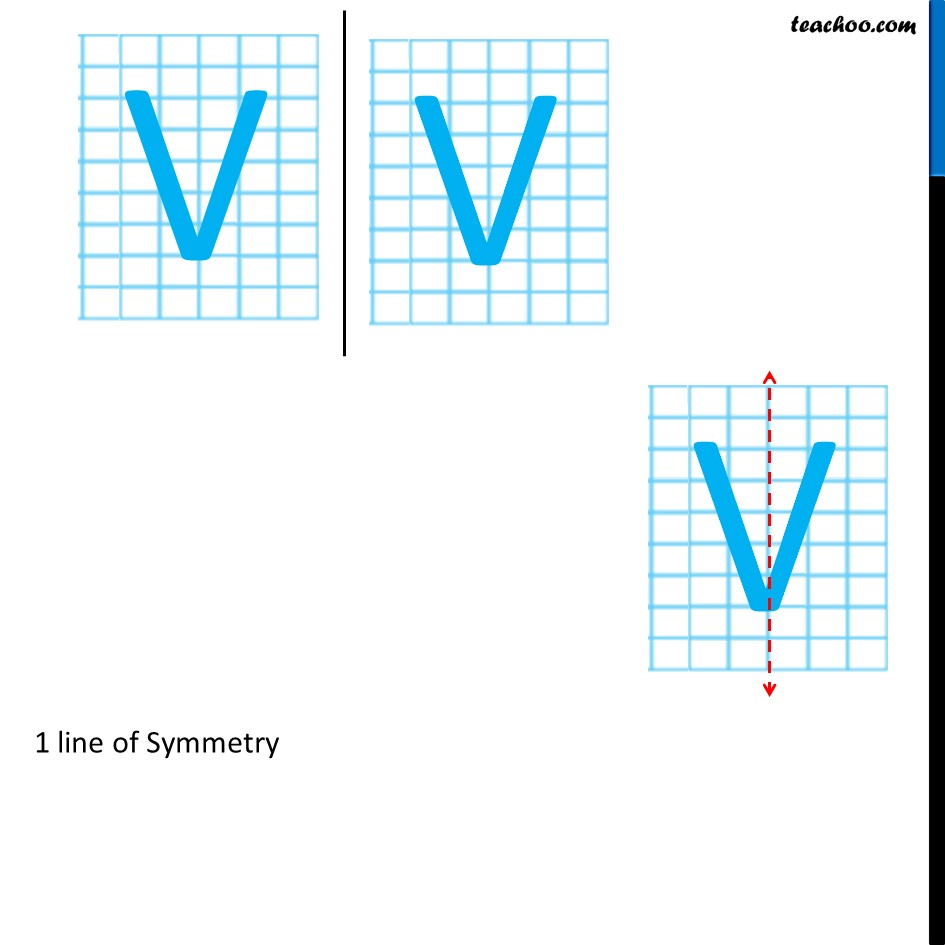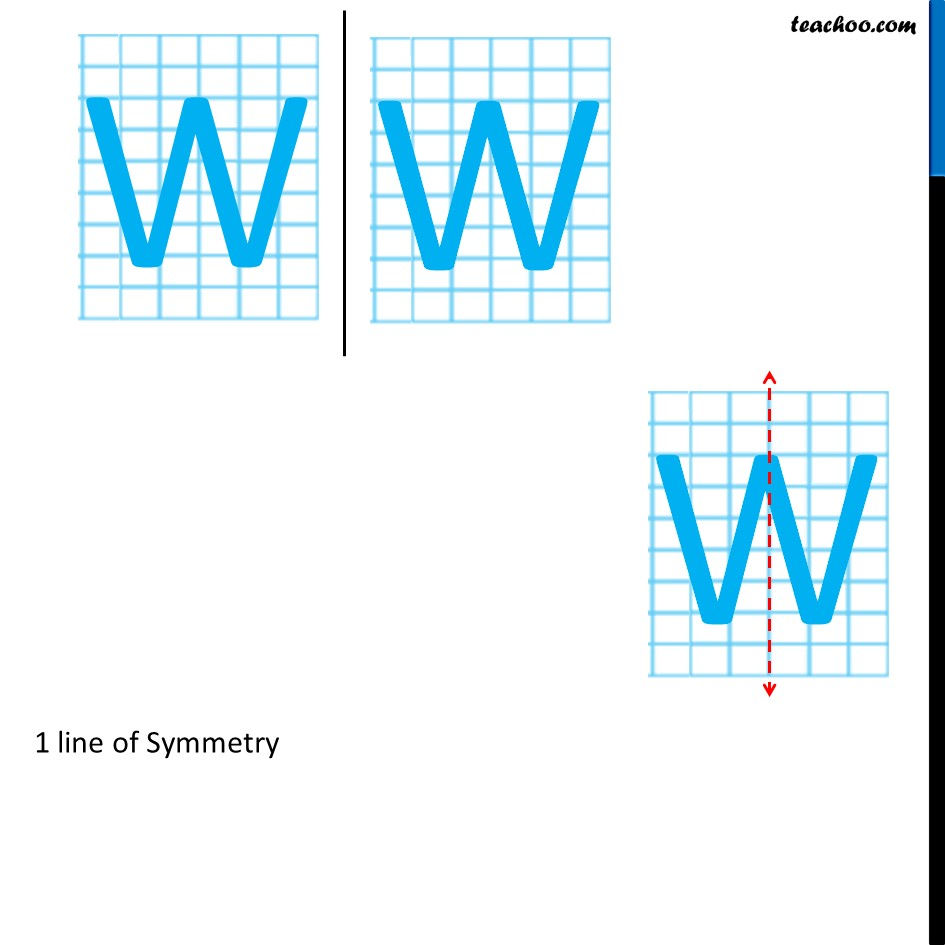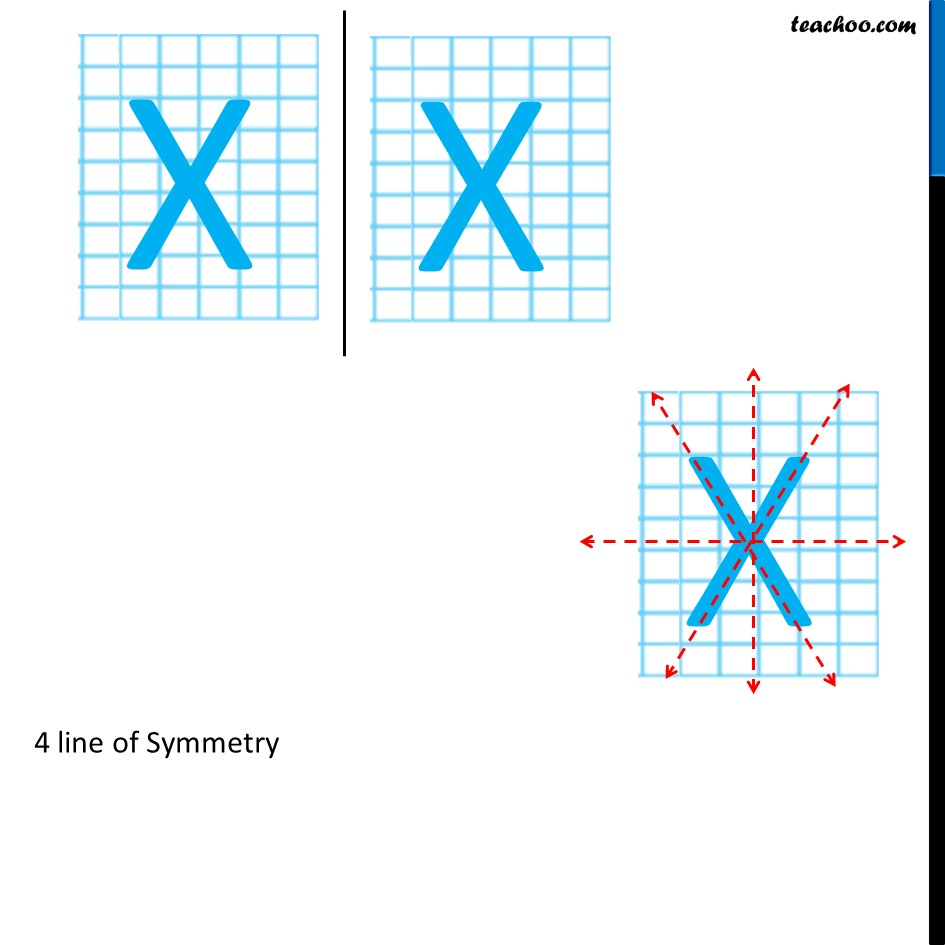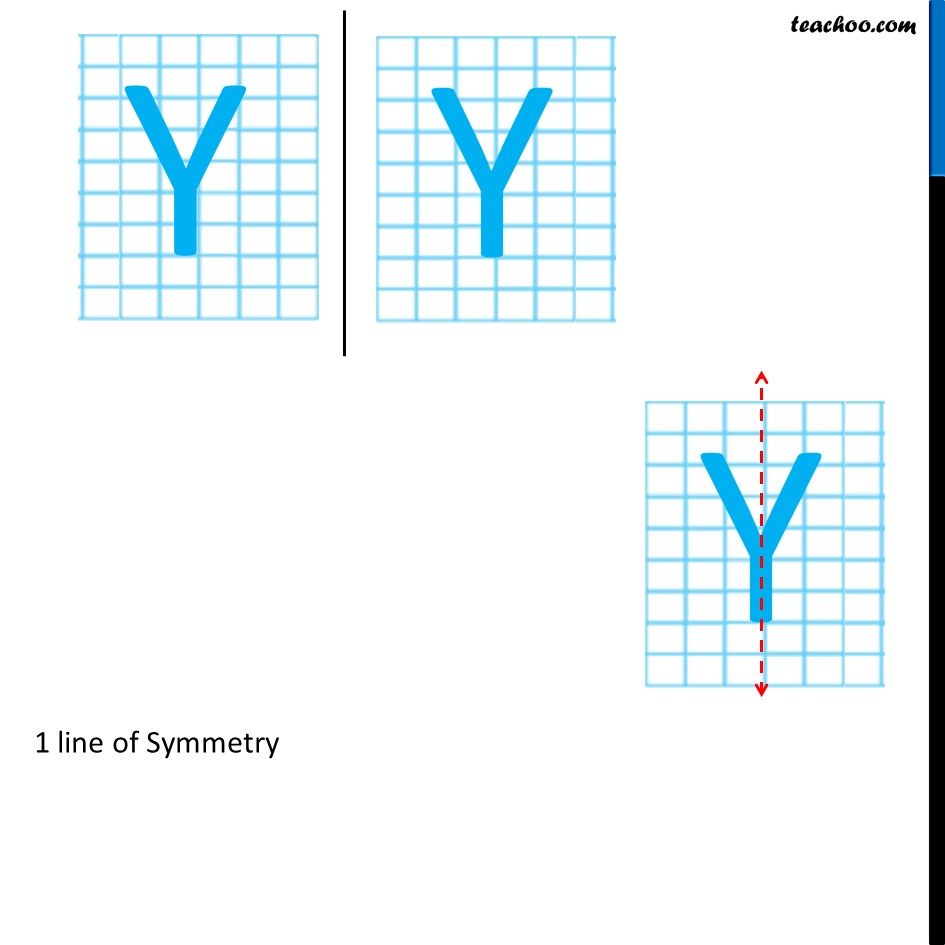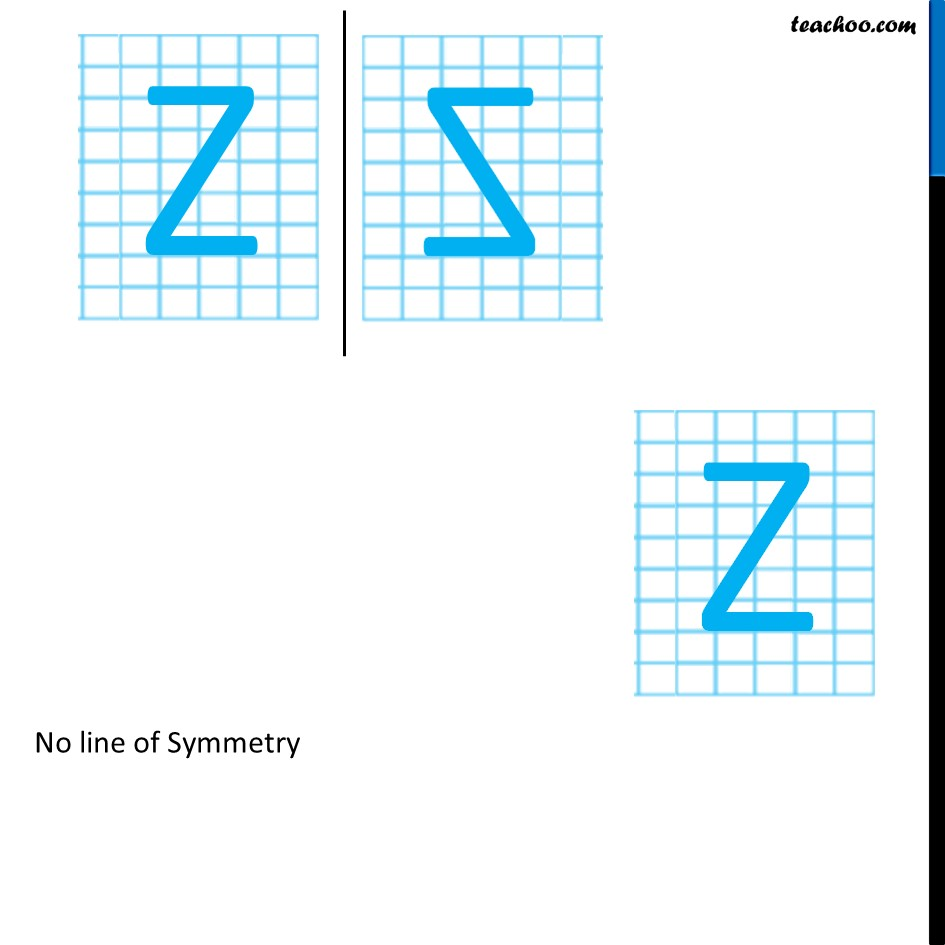1. Chapter 13 Class 6 Symmetry
2. Serial order wise
3. Ex 13.2

Transcript

Ex 13.2, 7 Consider the letters of English alphabets, A to Z. List among them the letters which have (a) vertical lines of symmetry (like A)1 line of Symmetry Ex 13.2, 7 Consider the letters of English alphabets, A to Z. List among them the letters which have (b) horizontal lines of symmetry (like B)1 line of Symmetry Ex 13.2, 7 Consider the letters of English alphabets, A to Z. List among them the letters which have (c) no lines of symmetry (like Q)1 line of Symmetry 1 line of Symmetry 1 line of Symmetry No line of Symmetry No line of Symmetry 2 line of Symmetry 2 line of Symmetry No line of Symmetry 1 line of Symmetry No line of Symmetry 1 line of Symmetry No line of Symmetry If we draw O like circle Infinite line of Symmetry If we draw O like oval 2 line of Symmetry If we draw O like oval 2 line of Symmetry If we draw O like oval 2 line of Symmetry No line of Symmetry No line of Symmetry No line of Symmetry cNo line of Symmetry No line of Symmetry 1 line of Symmetry 1 line of Symmetry 1 line of Symmetry 1 line of Symmetry 1 line of Symmetry No line of Symmetry

Ex 13.2

Chapter 13 Class 6 Symmetry
Serial order wise

About the AuthorDavneet Singh
Davneet Singh is a graduate from Indian Institute of Technology, Kanpur. He has been teaching from the past 10 years. He provides courses for Maths and Science at Teachoo.